# The frequency of higher rank in a family of quadratic twists

Random matrix theory can be used to predict the frequency of rank 2 curves in a family of quadratic twists, but it does not seem to be able to tell us what the prediction is for quadratic twists of rank 3 or higher.

The reason that the RMT approach fails is that the height of a generating point is a factor in the formula for the central value of the derivative of the L-function of a rank 1 curve. We don't have a prediction for the distribution of the heights of the generating points, and so can't predict (within the collection of curves of odd rank) the distribution the critical derivatives of the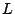-functions. Since the frequency of vanishing is determined by analyzing the tail of that distribution, we are unable to predict how often the derivative of the L-series is 0.

In [ arXiv:math/0010056] (to appear in Exp. Math.), Rubin and Silverberg show that for several infinite families of elliptic curves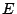, the number of quadratic twists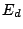,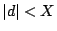, with rank at least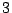is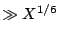. (Assuming the Parity Conjecture, the same result holds withreplaced by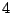.) Some of these examples were previously obtained by Stewart and Top [ MR 95m:11055].

Rubin and Silverberg ask whether it is possible to find a hyperelliptic curve over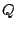whose jacobian contains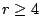copies of a fixed elliptic curve. This would give a lower bound for the frequency of quadratic twists ofof rank at least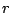.

Back to the main index for L-functions and Random Matrix Theory.